##### Geometry Chapter 8 Review

0
Set Details Share
created 6 years ago by kstedman
97 views
Includes all of the formulas for the Chapter 8 Review.
Page to share:
Embed this setcancel
COPY
code changes based on your size selection
Size:
X
1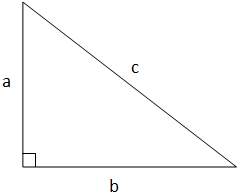a2 + b2 = c2

2x = 12

y = 10.39

3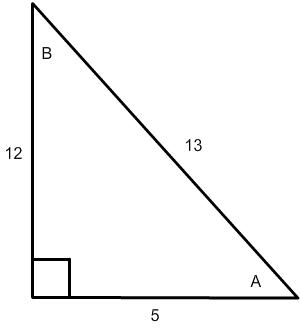What is sin A?

sin A = 12/13

4What is cos A?

cos A = 5/13

5What is tan A?

tan A = 12/5

6What is sin B?

sin B = 5/13

7What is cos B?

cos B = 12/13

8What is tan B?

tan B = 5/12

9What is the law of sines?

a = b = c

sin A sin B sin C

10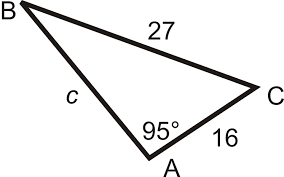Using the law of sines, what is the equation to solve for <B?

sin B = sin 95

16 27

11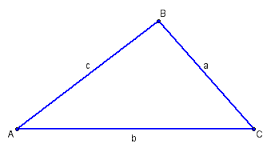If you are solving for a using the law of cosines, what formula do you use?

a2 = b2 + c2 - 2bc cos A

12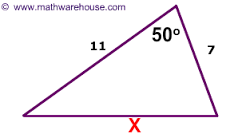If you are solving for x using the law of cosines, what formula do you use?

x2 = 72 + 112 - 2(7)(11)cos 50

13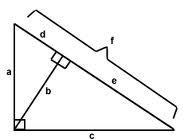Given the diagram, what is b equal to?

b = sqrt (d*e)

14Given the diagram, what is a equal to?

a = sqrt (d*f)

1516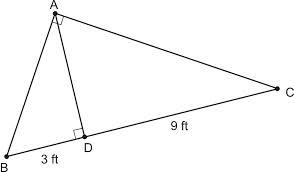Fiind AB.

AB = sqrt (3*12)

17Find AC.

AC = sqrt (9*12)